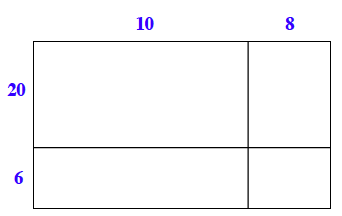### Home > CC1 > Chapter 3 > Lesson 3.2.1 > Problem3-96

3-96.

Rewrite each product below using the Distributive Property. Then simplify to find the answer. Homework Help ✎

1. $18(26)$

Complete the generic rectangle below to find the product.2. $6(3405)$

Write this as $6(3000 + 400 + 5)$.

Distribute the $6$ throughout the sum: $18000 + 2400 + 30 = 20430$

$20,430$

3. $21(35)$

This can be written as $(20 + 1)(30 + 5)$. Try using a generic rectangle, like the one in part (a), to find the product.